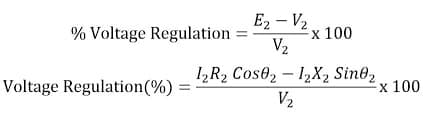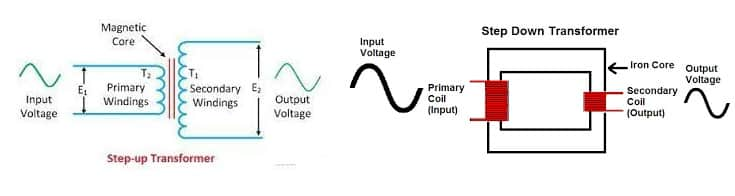# Transformer Interview Questions & Answers Part-2

What is the formula of transformer voltage regulation?A transformer has 300 turns on the primary, 100 turns on the secondary, and 120VAC applied to the primary, what is the voltage across the secondary?

V2=40 Volts

The same transformed can be used as step up or step down transformer. It all depends on which side voltage is being applied. It is very easy to identify the transformer for step up or step down operation.Measure the resistance of primary and secondary winding of the transformer.

The high voltage winding has more resistance because there is more number of turns of thinner cross section area wire.

The low voltage winding has low resistance because there is less number of turns of thicker cross section area wire.

Now we can use this transformer as a step or step down operation.

When transformer is on load, the system power factor can be improved to the some extent by lowering the voltage through OLTC. The decreased voltage reduce the reactive power, and thus power factor gets improved

It is generally recommended to use differential protection relay in 5 MVA and above rating transformers.

This can be done if equipment accepts increased frequency.

KVA load on Transformer is; =√3VI = 1.732 X 11x 100 = 1905.2 KVA

Conditions for Parallel Operation of Transformers

The following conditions must be satisfied for satisfactory performance when two or more transformers run in parallel.These are the conditions for parallel operation of transformers.

1. The Same voltage ratio of transformer
2. Same percentage impedance
3. Same Same polarity
4. The same phase sequence
5. Same frequency

Why is more energy lost in iron loss compared to copper loss throughout the day in a distribution transformer?

The distribution transformer is supposed to deliver the variable load.The iron loss will be consumed throughout the day as the transformer remains in operation continually, and the copper loss is variable and depends on the loading of the transformer.

The distribution transformer has more core loss as compared to the copper loss and that is why the transformer is designed for lesser iron loss. The all day efficiency is applicable for the distribution transformer.

It is possible to reduce the size of the transformer with an use of ferrite core. The higher frequency ac needs lower turns to get the same KVA as we get with many turns with lower frequency.

If supply is fed at the side of the transformer having more turns the transformer acts as a step down transformer.If supply is fed at the side of the transformer having less turns the transformer acts as a step up transformer.

For higher frequency application the soft core is used in the transformer to reduce the core losses.

6000/250= NP/Ns
24= NP/Ns
Turns ratio of transformer is 24:1

The turns ratio of a transformer is 10. What does it mean?

The turns ratio is the ratio of primary winding turns to secondary winding turns. Turns ratio= Np/Ns The high voltage side has more turns than the low voltage side of the transformer.

What is the use of silica gel in a power transformer?

Silica gel is used in the oil filled transformer to absorb the moisture from the air which enters in the conservator through the breather.The breather is filled with moisture absorbent (silica gel).

Which causes overheating of transformer?

The transformer overheats if

1. The transformer is operated above its rated flux density
2. Transformer is operated above its rated current capacity

In both the cases,the heat will be developed and it cause overheating of transformer. If the transformer is operated at its rated capacity the temperature rise can happen if cooling system is not properly working.

Related Posts